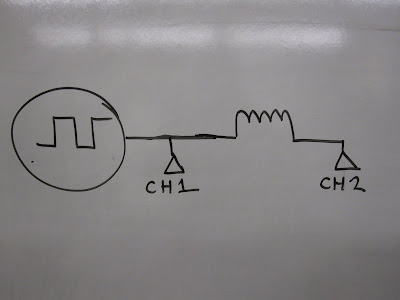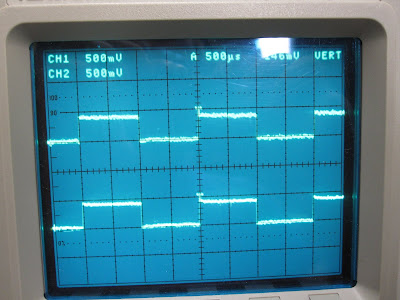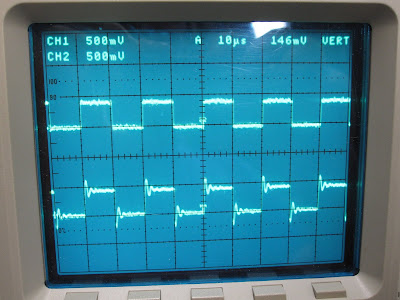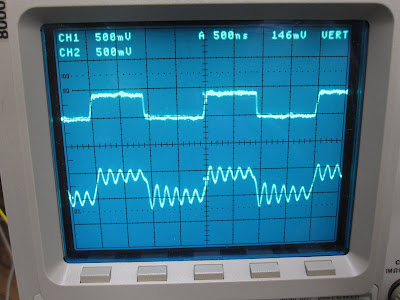## Coil Inductance

27 08 2011

Let’s take a look at the coil inductance.

A fun and easy place to start is look at some AC going through the coil (suggested in comments).

I used the oscilloscope’s test wave generator. I’m using two 10:1 probes connected thusly:Channel 1 is shown above channel 2 on these oscilloscope screens:

Not much distortion at  500Hz:At 50kHz we start to see some distortion:At 500kHz we have obvious distortion:Not sure what this tells us, but I’m sure it tell us something!

Now lets try a more abstract approach to the problem. We have a 6cm diameter air core coil with 60 turns. I found this handy inductance calculator. I looked up the thickness of 18 AWG wire and the relative permeability of air.Theoretically the coil has 0.56mH of inductance. Sound right?
Given inductance and frequency, you can calculate impedance…which is what we’re after. But what’s the frequency of a single pulse?

UPDATE: The rectangular function may be useful for calculating frequency.

### 17 responses

27 08 2011

The rectangle function represents a single pulse, whereas the square wave function (http://en.wikipedia.org/wiki/Square_wave) is a more accurate description of the test you’re doing.

Both the rectangle pulse and the square wave are a sum of many different frequency components, which you can find by taking the fourier transform of the function. The coil will present a different impedance to each frequency component, with progressively higher impedance for each of the higher frequency harmonics.

From the scope trace, it looks like the coil behaves more than just as a pure inductance. I think that the decaying ringing after every pulse is characteristic of an LRC circuit. Measuring the frequency of the excited rings ought to tell you L/C, and the decay time constant will tell you R. A pure inductance would just present an exponential rise and fall after each transition.

29 08 2011

The decaying ringing looks very much like the result if you’ve got a low bandpass filter. The result pulses are basically the square wave, fourier transformed, cut out a buncha high frequency stuff with a heaviside function, then inverse fourier it back.

Since you have an array of caps in parallel (IIRC) this totally makes sense, it looks like a canonical discrete low-pass filter. You may want to take the analytical solution of the RC filter equation and see if it matches up with the highest frequency in the graphs. Email me if you want me to give the math some effort.

http://en.wikipedia.org/wiki/RC_circuit

29 08 2011

LOL, you have an RL circuit, but that can still be a low-bandpass filter.

28 08 2011

I don’t know what you mean by “bottom shows signal through the coil.” Instrumentation detail would clarify.
Taking some kind of guess: I am almost certain that your present signals are due to the scope probe. It’s looks to me that you are using a 1:1 probe instead of a 10:1 . The 10:1 probes are better and have high frequency adjustment. Lacking that try a clip lead from the ground lug on the front of the scope to where ever you have the ground of the probe attached; actually a braid connection would be far better. The shorter the better; if I am right it’s the inductance of the scope probe ground that is fouling up the signal.

For the physical configuration you have I calculate a coil resistance of .237 ohms.
That gives an intrinsic time constant L/R of 2.3ms; which in fact is about what your high voltage readings are. So it’s reasonable to proceed to a CRLR type of waveform analysis. I will post the spice result later today. I think I will find that it matches your original test signals closely; allowing for extra resistance here and there. That leaves the spike. I know you mentioned it somewhere but what is the part number of the thyristor? Just curiosity.
Actually your waveforms are making more and more sense to me.

Ray

28 08 2011

Ray, I updated the post for clarity.

Probes are 10:1

I’m using this SCR for triggering: https://prometheusfusionperfection.com/2010/03/27/new-arrivals-6/

28 08 2011

Here’s a plot of an analytical solution to a simple RLC circuit that I *think* is representative of what you are doing; python script to make the plot here. I think you should be able to fit your current trace by changing the L parameter (since you’ve measured R and C). This will give you an estimate of the inductance.

28 08 2011

Sweet thanks!

28 08 2011

No problem; I updated the python script to fit one of the traces from your previous post. Here’s a plot of the fit with the estimated parameters.

28 08 2011

Whoa!

g3data is sweet!

http://www.frantz.fi/software/g3data.php

Is there a mac build of g3data?

28 08 2011

not sure about Mac; it’s in lots of linux distros so it shouldn’t be too hard to build

it is really great for getting some quick and dirty estimates; especially from old reports that might just have plots, but no tables

28 08 2011

Here is a spice circuit, listing and plot; in case anybody else wants to run circuit. The net-list format is for LTspice from linear technology.
I would appreciate it if you would review the values (or the circuit) and correct them; since my waveform doesn’t conform to well. I will rerun if you want. That’s the nice thing about simulations; once set up they can be run over and over.
Also note that there is no reason in this circuit for the spike on some of your pictures; since that allows large currents I would suspect the inductance failing. Either shorted winding or interaction with plasma.
The failure of inductance can be tested with a pickup coil to see if the magnetic field actually goes up at that time.

Ray

Wave form:

28 08 2011

Ray: awesome work!

I’ll try and get this spice model running myself.

28 08 2011

Theoretically the coil has 0.56mH of inductance…
Not exactly, 6 groups of 10 turns is not the same as 1 of 60 turns. If you put 10 turns into your calculator and then multiply it by 6 (in series) it will give you a smaller result (closer to real) but possibly not hugely different.
The lead in wires will also add to the inductance.
The ringing you see is due to some capacitance , possibly from the coil to the chamber and can be calculated from the frequency once you know the exact inductance.

29 08 2011

The inductance of a (multiple turn) wire loop depends on N^2 (N = the number of turns) so it’s a big difference between 1 loop with 60 turns and 6 loops with 10 turns. If they were totally independent the coils would be about 100 μH, but they are definitely close enough for mutual inductance to change that number (no idea of how much though)…

30 08 2011

No, you don’t need capacitance see ringing. a simple RL circuit will act as a low-bandpass filter, and the ringing is just the sum of some higher harmonics of the square wave. You see ringing in an RLC circuit if your power supply is DC, but we’re working with AC here.

For an RL circuit, the bandpass is R/(2piL).

29 08 2011

(My comment disappeared, trying again…)

The inductance of a (multiple turn) wire loop depends on N^2 (N = number of turns) so the inductance of 1 loop with 60 turns is not the same as 6 loops with 10 turns. If the coils were totally independent the total inductance would be about 100 μH.
The coils are definitely close enough for mutual inductance to change that number, but I’m not sure exactly how… (does the opposing coils increase or decrease the inductance?)

31 08 2011

Opposing coils will reduce the inductance, in much the same way a twisted pair or coax has low inductance, the forward and return currents “fields” are in opposite directions. Power to the coils forward and return should be twisted for this reason, ie to reduce “leakage inductance”. BTW the wires will try to push against each other, possibly causing some movement under current pulses.